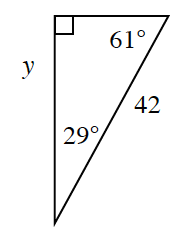### Home > INT2 > Chapter 4 > Lesson 4.2.2 > Problem4-73

4-73.Eugene wants to use the cosine ratio to calculate $y$ for the triangle below.

1. Which angle should he use to write an equation and solve for $y$ using the cosine ratio? Why?

$\text{cos } \theta =\frac{\text{adj.}}{\text{hyp.}}$

The side labeled $y$ is adjacent (next to) which angle?

2. Set up an equation, and solve for $y$ using cosine.

$\cos(\text{?})=\frac{y}{42}$